# 老周：10.10期货交易策略 ... 经济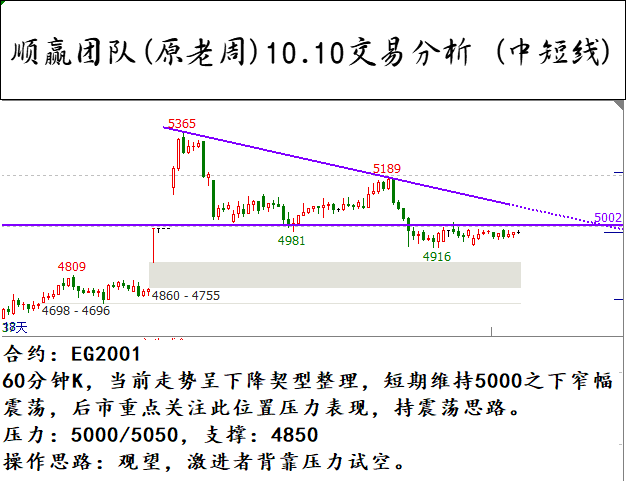500)this.width=500' align='center' hspace=10 vspace=10 rel='nofollow'/>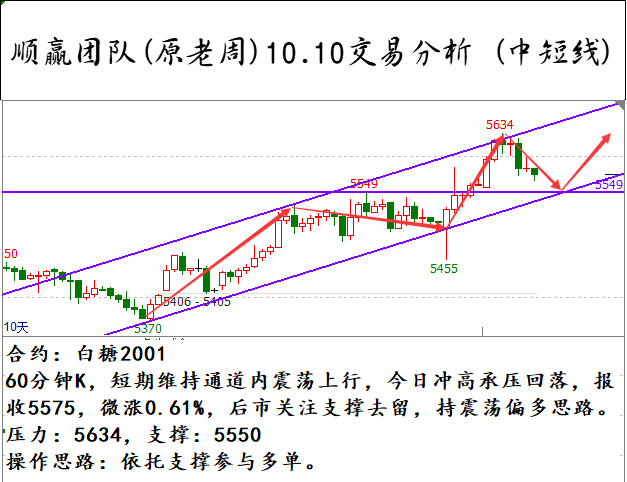500)this.width=500' align='center' hspace=10 vspace=10 rel='nofollow'/>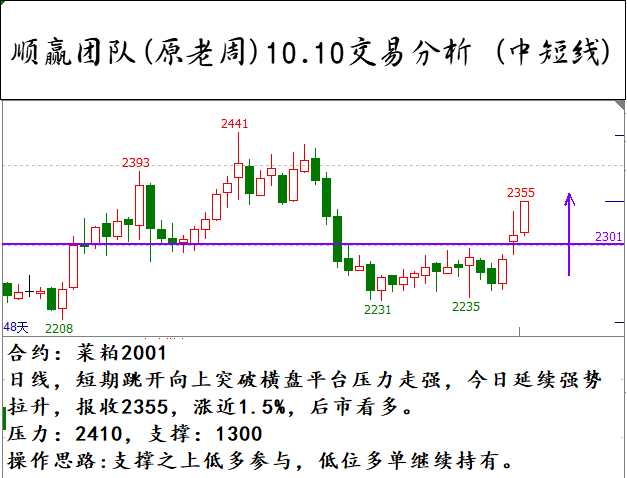500)this.width=500' align='center' hspace=10 vspace=10 rel='nofollow'/>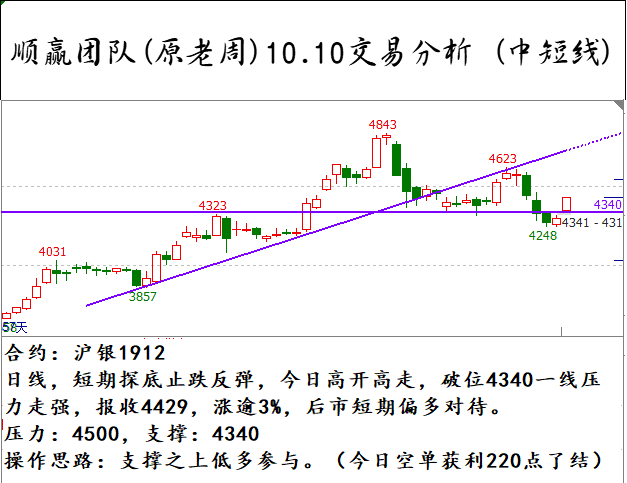500)this.width=500' align='center' hspace=10 vspace=10 rel='nofollow'/>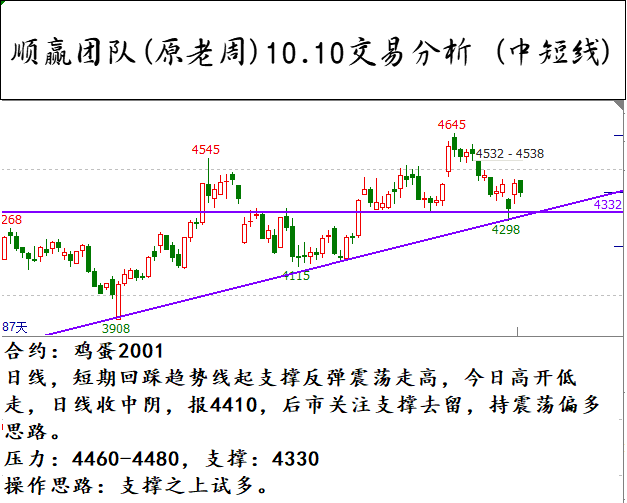500)this.width=500' align='center' hspace=10 vspace=10 rel='nofollow'/>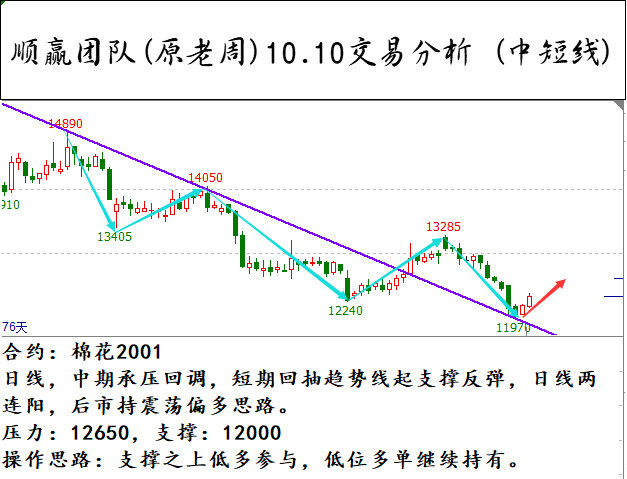500)this.width=500' align='center' hspace=10 vspace=10 rel='nofollow'/>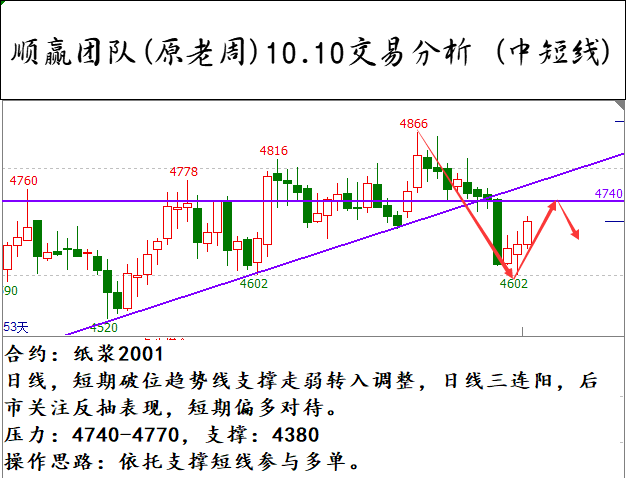500)this.width=500' align='center' hspace=10 vspace=10 rel='nofollow'/>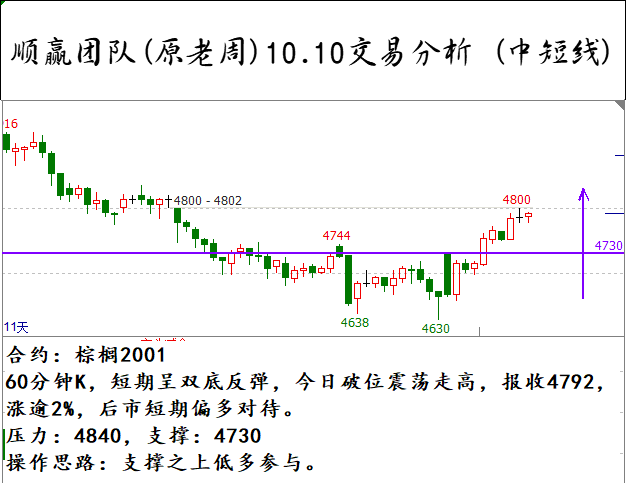500)this.width=500' align='center' hspace=10 vspace=10 rel='nofollow'/>

60分钟K，短期呈双底反弹，今日破位震荡走高，报收4792，涨逾2%，后市短期偏多对待。

60分钟K，短期维持通道内震荡上行，今日冲高承压回落，报收5575，微涨0.61%，后市关注支撑去留，持震荡偏多思路。

60分钟K，当前走势呈下降契型整理，短期维持5000之下窄幅震荡，后市重点关注此位置压力表现，持震荡思路。

•••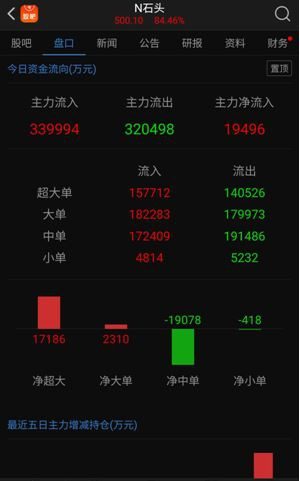•